The NLMIXED Procedure

## Example 61.4 Poisson-Normal Model with Count Data

This example uses the pump failure data of Gaver and O’Muircheartaigh (1987). The number of failures and the time of operation are recorded for 10 pumps. Each of the pumps is classified into one of two groups corresponding to either continuous or intermittent operation. The data are as follows:

```   data pump;
input y t group;
pump = _n_;
logtstd = log(t) - 2.4564900;
datalines;
5  94.320 1
1  15.720 2
5  62.880 1
14 125.760 1
3   5.240 2
19  31.440 1
1   1.048 2
1   1.048 2
4   2.096 2
22  10.480 2
;
```

Each row denotes data for a single pump, and the variable logtstd contains the centered operation times.

Letting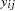denote the number of failures for theth pump in theth group, Draper (1996) considers the following hierarchical model for these data: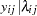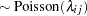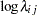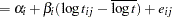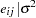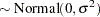The model specifies different intercepts and slopes for each group, and the random effect is a mechanism for accounting for overdispersion.

The corresponding PROC NLMIXED statements are as follows:

```   proc nlmixed data=pump;
parms logsig 0 beta1 1 beta2 1 alpha1 1 alpha2 1;
if (group = 1) then eta = alpha1 + beta1*logtstd + e;
else eta = alpha2 + beta2*logtstd + e;
lambda = exp(eta);
model y ~ poisson(lambda);
random e ~ normal(0,exp(2*logsig)) subject=pump;
estimate 'alpha1-alpha2' alpha1-alpha2;
estimate 'beta1-beta2' beta1-beta2;
run;
```

The selected output is as follows.

Output 61.4.1 Dimensions Table for Poisson-Normal Model
The NLMIXED Procedure

Dimensions
Observations Used 10
Observations Not Used 0
Total Observations 10
Subjects 10
Max Obs Per Subject 1
Parameters 5

The "Dimensions" table indicates that data for 10 pumps are used with one observation for each (Output 61.4.1).

Output 61.4.2 Iteration History for Poisson-Normal Model
Iteration History
Iter   Calls NegLogLike Diff MaxGrad Slope
1   2 30.6986932 2.162768 5.107253 -91.602
2   5 30.0255468 0.673146 2.761738 -11.0489
3   7 29.726325 0.299222 2.990401 -2.36048
4   9 28.7390263 0.987299 2.074431 -3.93678
5   10 28.3161933 0.422833 0.612531 -0.63084
6   12 28.09564 0.220553 0.462162 -0.52684
7   14 28.0438024 0.051838 0.405047 -0.10018
8   16 28.0357134 0.008089 0.135059 -0.01875
9   18 28.033925 0.001788 0.026279 -0.00514
10   20 28.0338744 0.000051 0.00402 -0.00012
11   22 28.0338727 1.681E-6 0.002864 -5.09E-6
12   24 28.0338724 3.199E-7 0.000147 -6.87E-7
13   26 28.0338724 2.532E-9 0.000017 -5.75E-9

 NOTE: GCONV convergence criterion satisfied.

The "Iteration History" table indicates successful convergence in 13 iterations (Output 61.4.2).

Output 61.4.3 Fit Statistics for Poisson-Normal Model
Fit Statistics
-2 Log Likelihood 56.1
AIC (smaller is better) 66.1
AICC (smaller is better) 81.1
BIC (smaller is better) 67.6

The "Fit Statistics" table lists the final log likelihood and associated information criteria (Output 61.4.3).

Output 61.4.4 Parameter Estimates and Additional Estimates
Parameter Estimates
Parameter Estimate Standard Error DF t Value Pr > |t| Alpha Lower Upper Gradient
logsig -0.3161 0.3213 9 -0.98 0.3508 0.05 -1.0429 0.4107 -0.00002
beta1 -0.4256 0.7473 9 -0.57 0.5829 0.05 -2.1162 1.2649 -0.00002
beta2 0.6097 0.3814 9 1.60 0.1443 0.05 -0.2530 1.4724 -1.61E-6
alpha1 2.9644 1.3826 9 2.14 0.0606 0.05 -0.1632 6.0921 -5.25E-6
alpha2 1.7992 0.5492 9 3.28 0.0096 0.05 0.5568 3.0415 -5.73E-6

The "Parameter Estimates" and "Additional Estimates" tables list the maximum likelihood estimates for each of the parameters and two differences (Output 61.4.4). The point estimates for the mean parameters agree fairly closely with the Bayesian posterior means reported by Draper (1996); however, the likelihood-based standard errors are roughly half the Bayesian posterior standard deviations. This is most likely due to the fact that the Bayesian standard deviations account for the uncertainty in estimating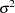, whereas the likelihood values plug in its estimated value. This downward bias can be corrected somewhat by using the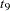distribution shown here.